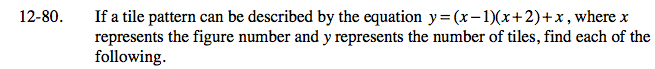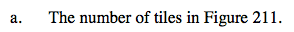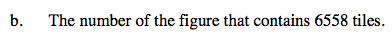### Home > CA > Chapter Ch12 > Lesson 12.4.2 > Problem12-80

12-80.
1. If a tile pattern can be described by the equation y = (x − 1)(x + 2) + x, where x represents the figure number and y represents the number of tiles, find each of the following. Homework Help ✎

1. The number of tiles in Figure 211.

2. The number of the figure that contains 6558 tiles.Substitute 211 into the equation for x.Substitute 6558 into the equation for y.

6558 = (x − 1)(x + 2) + x

6558 = x² + x − 2 + x

6558 = x² + 2x + 2

Set the equation equal to zero.

0 = x² + 2x − 6556

Factor the expression.

0 = (x + 82)(x − 80)

x = −82 or 80

The figure number cannot be negative.

Figure 80